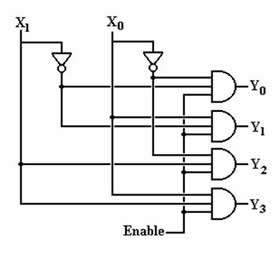# Difference between Demultiplexer and Decoder

##### Key Difference: A demultiplexer or DMUX is a combination circuit that contains one data input, few control inputs and many outputs, whereas a decoder is a logic circuit that converts a binary number to its equivalent decimal number.

To understand the demultiplexer and decoders the concept of combinational circuits must be clear. Combinational logic circuits are defined by the logical function of their current input state. The state can be either 0 or 1, at any instance. In a combinational logic circuit,  the output is dependent on the combination of its inputs. If in any case the change in a state of input occurs, then the output is also affected by the change. These circuits are basically made up from three basic gates:

1. AND
2. OR
3. NOT

These gates are combined to produce simple or complicated switching circuits. The function of the combinational logic circuit can easily be obtained by implementation of Boolean algebra, truth table or by logic diagrams. These combinational logic circuits are classified into three broad categories:

1. Arithmetic and logical functions
2. Data Transmission
3. Code Converters

The data transmissions category includes the multiplexers, demultiplexers, encoders and decoders. Thus, this particular category is of great importance in terms of defining the differences between the demultiplexer and decoder.A demultiplexer is a circuit that receives the information on its single line and then transmits the information on any one of the possible 2^n output lines. Demultiplexers are used to implement logic functions.

A decoder is also a kind of combinational circuit that has multiple inputs and multiple outputs, and used for conversion of coded inputs into the coded outputs. The bits in the input code are generally less than the bits that are present in the output code. A decoder basically decodes an input code. For example, if a binary code of n bits is provided to the decoder, then the decoder may tell that which code is this from the possible 2^n codes. A decoder can also be defined as a device that does just the reverse of an encoder.Thus, we can define the differences between a decoder and a demultiplexer by considering these two important factors. A decoder is a many input to many output device, whereas demultiplexers is a one input to many outputs device. Another difference between them can be expressed as there are no selection lines in decoder, whereas in the case of a demultiplexer, the selection of specific outline is controlled by the value of selection lines.

The differences can be summed as:-

 Demultiplexer Decoder Definition 1 data input 2^n outputs It has n inputs 2^n outputs It has n control inputs Characteristic Connects the data input to the data output Selects one of the 2^n outputs by decoding the binary value on the basis of n inputs Reverse of Multiplexer Encoder

Images Courtesy: electronics-tutorials.ws

Where n is any +ve integer.. 1,2,3,4,.... By this silly qstn I can understood that u don't anything about switching theory & logic design

n stands for selection lines by which output is designed

n -select lines, if n=3, then 2^3 outputs are possible, but in DEMUX single input only available.

It is Usefull

there's a note in the table of differences of DEMUX AND DECODER the colums must be reverse !

Yes...
But not for the last field

Thank you SS for taking an interest in the article, but the changes were already made earlier. A demultiplexer takes a single input signal and selects one among the many output lines. A decoder like line decoder takes n digital binary number and decodes it into 2^n lines. Demultiplexers connect single source to one of the many multiple destination lines. Multiplexer is the exact opposite of a demultiplexer, whereas encoder is the exact opposite of decoder.